Surfaces

Start this free course now. Just create an account and sign in. Enrol and complete the course for a free statement of participation or digital badge if available.

Free course

# 4.6.1 Remarks

1. We already know that the characteristic numbers are topological invariants, that is, any two homeomorphic surfaces have the same values for the characteristic numbers. Thus it is solely the converse, namely if two surfaces have the same values for the characteristic numbers then they are homeomorphic, that we have to prove.

2. It follows from the Classification Theorem that if we restrict our attention to orientable surfaces (ω = 0), then the numbers β and χ characterise the surface completely – and similarly if we restrict our attention to non-orientable surfaces (ω = 1).

3. Our assumption that all surfaces are compact guarantees that the characteristic numbers are finite,

4. The Classification Theorem allows us to refer to the sphere, the torus, the Klein bottle, etc., rather than a sphere, a torus and a Klein bottle, etc.

## Problem 24

Which surfaces are characterised by the following sets of characteristic numbers?

1.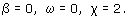2.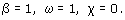3.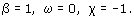4.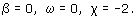5.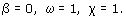1. Sphere.

2. Möbius band.

3. Torus with 1 hole.

4. 2-fold torus.

5. Projective plane.

## Problem 25

Find the characteristic numbers of the surface obtained by gluing two Möbius bands along their boundaries. Deduce from the Classification Theorem that this surface is homeomorphic to the Klein bottle.

The surface obtained by gluing two Möbius bands together has no boundary components (β = 0), and is non-orientable (ω = 1). Also, by Theorem 13, its Euler characteristic is the sum of the Euler characteristics of each separate surface, so χ = 0 + 0 = 0. The Klein bottle has these values for its characteristic numbers, so (by the Classification Theorem) the glued surface is homeomorphic to the Klein bottle.

## Worked problem 4

Find the characteristic numbers of the closed disc with two straps, shown in Figure 97.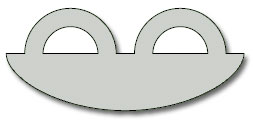Figure 97

### Solution

The surface has three boundary components, so β = 3. The surface contains no Möbius band, and is therefore orientable, so ω = 0. To find the Euler characteristic, we choose a subdivision. The one shown in Figure 98 has 8 vertices, 12 edges and 3 faces, so χ = 8 − 12 + 3 = −1.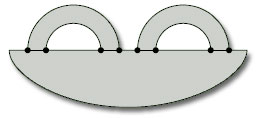Figure 98

You could also argue that, as the surface is a closed disc with two holes, its Euler characteristic is 1 − 2= −1 (by Theorem 11).

## Problem 26

Consider the closed disc with two straps added in other ways, sometimes with a twist, as shown in Figure 99. Compute the characteristic numbers of each surface, and then use the Classification Theorem to determine which of these surfaces are homeomorphic.

Each surface has a subdivision with 8 vertices, 12 edges and 3 faces, as indicated in Worked Problem 4. The Euler characteristic of each surface is therefore −1, as before.

The characteristic numbers β and ω are as follows.

 surface S1 S2 S3 S4 S5 β 1 1 2 1 2 ω 0 1 1 1 1

It follows from the Classification Theorem that the surfaces S2 and S4 are homeomorphic, as are the surfaces S3 and S5; no other two surfaces are homeomorphic.

## Problem 27

For each of the following paper surfaces, mark a subdivision and hence determine the characteristic numbers of the surface.

(a) We have drawn a subdivision with 12 vertices.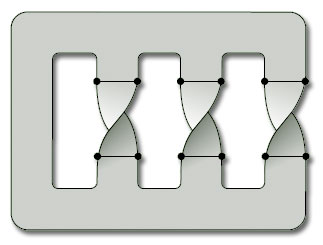There are 18 edges and 4 faces, so the Euler characteristic is χ = 12 − 18 + 4 = −2.

There is one boundary component, so β = 1.

The surface is non-orientable (see Worked Problem 2(b)), so ω = 1.

(b) We have drawn a subdivision with 16 vertices.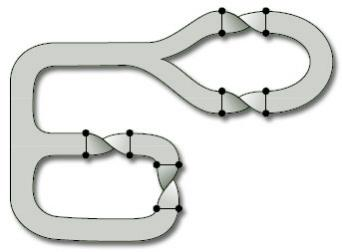There are 24 edges and 7 faces, so the Euler characteristic is χ = 16 − 24 + 7= −1.

There are three boundary components, so β = 3.

The surface is orientable (see Solution 14(b)), so ω = 0.

For future reference, we conclude this section with a table of some common surfaces with their characteristic numbers.

surfaceβωχ
sphere002
n-fold torus002 − 2n
Klein bottle010
projective plane011
closed disc101
cylinder200
Möbius band110

In addition, adding a hole to any surface increases its boundary number β by 1, keeps its orientability number ω the same, and decreases its Euler characteristic χ by 1.

M338_1